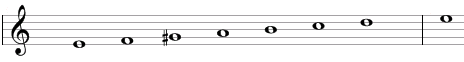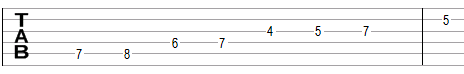# Spanish gypsy scale

The Spanish gypsy scale is a heptatonic scale that can be built using the following steps between notes: ½, 1½, ½, 1, ½, 1, 1.

One example of the scale is E, F, G#, A, B, C, D. This example is shown below in traditional notation and in guitar tablature notation.The Spanish gypsy scale is the fifth mode of the harmonic minor scale, which itself has the steps 1, ½, 1, 1, ½, 1½, ½. The harmonic minor scale in the example above would be A, B, C, D, E, F, G# (and itself is the natural minor scale, but with a raised seventh G# instead of G).

Another way to think about the Spanish gypsy scale is to take the Phrygian scale and raise its third. One example of the Phrygian is E, F, G, A, B, C, D and with a raised third this scale becomes E, F, G#, A, B, C, D. The Phrygian is commonly used in flamenco, where alternation between the minor and major third (G and G# in this example) is allowed to accommodate the major E chord (the major tonic triad) in the Phrygian. Thus, the scale carries the name "Spanish gypsy scale".

## Modes of the Spanish gypsy scale

The fourth mode of the Spanish gypsy scale is the harmonic minor scale. The harmonic minor scale in the example above would be A, B, C, D, E, F, G#.

## Three-note chords on the Spanish gypsy scale

The following are common triads built on the notes of the Spanish gypsy scale.

• On the root of the scale (on the tonic): major chord (e.g., E composed of E, G#, B), augmented chord (Eaug = E, G#, C) or suspended chord (Esus4 = E, A, B).
• On the second note (on the supertonic): major chord (F = F, A, C), minor chord (Fm = F, G#, C), or diminished chord (Fdim = F, G#, B).
• On the third note (on the mediant): augmented chord (G#aug = G#, C, E) or diminished chord (G#dim = G#, B, D).
• On the fourth note (on the subdominant): minor chord (Am = A, C, E) or suspended chords (Asus4 = A, D, E and Asus2 = A, B, E).
• On the fifth note (on the dominant): diminished chord (Bdim = B, D, F).
• On the sixth note (on the submediant): augmented chord (Caug = C, E, G#).
• On the seventh note (on the leading tone: minor chord (Dm = D, F, A), diminished chord (Ddim = D, F, G#) or suspended chord (Dsus2 = D, E, A).

## Four-note chords on the Spanish gypsy scale

The following are seventh chords built on the notes of the Spanish gypsy scale.

• On the first note: dominant seventh chord (e.g. E7 composed of E, G#, B, D) or augmented seventh chord (dominant seventh chord with a sharp fifth, E7#5 = E, G#, C, D).
• On the second note: major seventh chord (Fmaj7 = F, A, C, E), half-diminished seventh chord (F*7 = F, G#, B, D), or minor-major seventh chord (Fmmaj7 = F, G#, C, E).
• On the third note: half-diminished seventh chord (G#*7 = G#, B, D, F).
• On the fourth note: minor-major seventh chord (Ammaj7 = A, C, E, G#).
• On the fifth note: half-diminished seventh chords (minor seventh chord with a flat fifth, Bm7b5 = B, D, F, A or B*7 = B, D, F, G#).
• On the sixth note: augmented major seventh chord (Cmaj7#5 = C, E, G#, B).
• On the seventh note: minor seventh chord (Dmin7 = D, F, A, C), half-diminished seventh chord (Dm7b5 = D, F, G#, C), and diminished seventh chord (D*7 = D, F, G#, B).

## Intervals on the Spanish gypsy scale

The Spanish gypsy scale is composed of the following intervals.

• A minor second, e.g., the interval between E and F is equal to one semitone.
• A major third, e.g., the interval between E and G# is equal to four semitones.
• A perfect fourth, e.g., the interval between E and A is equal to five semitones.
• A perfect fifth, e.g., the interval between E and B is equal to seven semitones.
• A minor sixth, e.g., the interval between E and C is equal to eight semitones.
• A minor seventh, e.g., the interval between E and D is equal to ten semitones.

Scale, Scale (index)

### Filtered HTML

• Freelinking helps you easily create HTML links. Links take the form of [[indicator:target|Title]]. By default (no indicator): Click to view a local node.